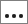# Define a Discrete Input Variable and Set the Number of Points

A discrete variable is an input variable that can take values from an orderable finite list of numeric values, for example x = 0.1, 0.2, 0.3, or 0.4.

This example assumes that you already have an input variable created.
1. In the Define Input Variables step, click the Modes tab.
2. In the Mode column for the input variable you are defining, select Discrete.
Note: The Values cell turns orange, which indicates you can edit its values.
3. In the Values cell, click.
4. In the dialog, edit the discrete value set and then click OK.
• To add content to this set, click Add Row; to remove content from this set, click Remove Row.
• To divide the value range into equal segments, change the lower and upper bounds, enter a value in the Step Number field, and then click Set.
• To create a set with equal step sizes, change the lower and upper bounds, enter a value in the Step Size field, and then click Set.